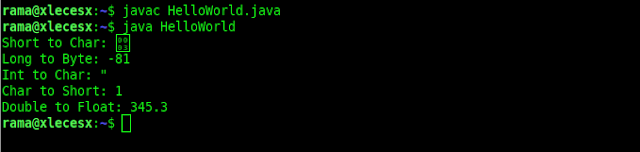# Casting And Type Conversion in JavaIn the Java programming language, Type Casting is used to determine and change a value from one data type to another data type. For example, if we have variable A with Integer data type, then we can determine the value of variable A to be float data type or vice versa. There are 2 types of Type Casting in Java called Implicit Casting and Explicit Casting.

Both are used to change the value of one data type variable. The difference between the two types is, Implicit is used to change from small data types to large data types, for example, Bytes to Long or Short to Double.

### Widening Conversion (Implicit Casting)

Implicit Casting is used to convert values from small data types to large data types, such as the following:
• From short data types to int, long, float, or double data types
• From int data types into long, float, or double data types
• From char data types into int, long, float, or double data types
• From float data types into double data types
• From long data types or into float or double data types
• From byte data type to short, int, long, float, or double data types
Example of an Implicit Casting program in Java:

``````
public class HelloWorld {
public static void main(String[] args){

short data1 = 457;
double double_data = data1; //short to double

char data2 = 'W';
long long_data = data2;//char to long

int  data3 = 456;
float int_data = data3;// int to float

byte data4 = 127;
long long_data2 = data4;//byte to long

float data5 = 565.3f;
double double_data2 = data5;//float to double

System.out.println("Short to Double: "+ double_data);
System.out.println("Char to Long: "+long_data);
System.out.println("Int to Float: "+int_data);
System.out.println("Byte to Long: "+long_data2);
System.out.println("Float to Double: "+double_data2);
}
}
``````

Result:### Narrow Conversion (Explicit Casting)

Explicit Casting is the opposite of Implicit Casting, which is to change values from large data types to small data types, such as:
• From short data types to bytes or char
• From double data types to bytes, short, char, int, long or float
• From char data types to bytes or short
• From long data types to bytes, short, char or int
• From int data types to bytes, short, or char
• From float data type to byte, short, char, int or long
• From byte data type into the char data type

Examples of Explicit Casting programs in Java:

``````
public class HelloWorld {
public static void main(String[] args){

short data1 = 3;
char char_data = (char) data1; //short to char

long data2 = 246447;
byte byte_data = (byte) data2;//long to byte

int  data3 = 34;
char char_data2 = (char) data3;// int to char

char data4 = 1;
short short_data2 = (short) data4;//char to short

double data5 = 345.3;
float float_data2 = (float) data5;//double to float

System.out.println("Short to Char: "+ char_data);
System.out.println("Long to Byte: "+byte_data);
System.out.println("Int to Char: "+char_data2);
System.out.println("Char to Short: "+short_data2);
System.out.println("Double to Float: "+float_data2);
}
}
``````

Result:What is important in the process of casting and conversion in Java is known beforehand about the type of data in java.